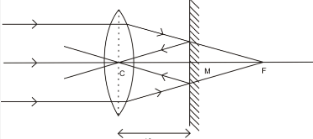# The distance between a convex lens and a plane mirror is 10 cm

The distance between a convex lens and a plane mirror is 10 cm. The parallel rays incidenton the convex lens after reflection from the mirror form an image at the optical centre of the lens. Find the focal length of the lensThe parallel rays incident on the convex lens converge at the focus,F of the lens. At the mirror, after reflection forms an image at the optical centre of the lens, C.
Thus, the image of the virtual point object F is formed at C.
f = CF
=CM + MF
= 10 + 10
= 20 cm
Thus, the focal length of the lens is 20 cm.